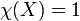# Space with Euler characteristic one

This article defines a property of topological spaces: a property that can be evaluated to true/false for any topological space|View a complete list of properties of topological spaces

## Definition

Suppose$X$ is a topological space that is a space with finitely generated homology, i.e., it has only finitely many nontrivial homology groups and all of them are finitely generated. We say that$X$ is a space with Euler characteristic one if the Euler characteristic of$X$ equals$1$, i.e.,$\chi(X) = 1$.

## Relation with other properties

### Stronger properties

Property Meaning Proof of implication Proof of strictness (reverse implication failure) Intermediate notions
contractible space homotopy-equivalent to a point (via acyclic) (via acyclic) Acyclic space|FULL LIST, MORE INFO
weakly contractible space weakly homotopy-equivalent to a point (via acyclic) (via acyclic) Acyclic space|FULL LIST, MORE INFO
acyclic space all the homology groups are zero, except for the zeroth homology group (via rationally acyclic) (via rationally acyclic) |FULL LIST, MORE INFO
space with finitely generated homology that is also a rationally acyclic space all the homology groups over the rationals, except the zeroth homology group, are zero. Equivalently, all integral homology groups except the zeroth one are torsion rationally acyclic and finitely generated homology implies Euler characteristic one Euler characteristic one not implies rationally acyclic

### Weaker properties

Property Meaning Proof of implication Proof of strictness (reverse implication failure) Intermediate notions
space with finitely generated homology finitely many nonzero homology groups, and they are all finitely generated |FULL LIST, MORE INFO
space with homology of finite type all homology groups are finitely generated |FULL LIST, MORE INFO